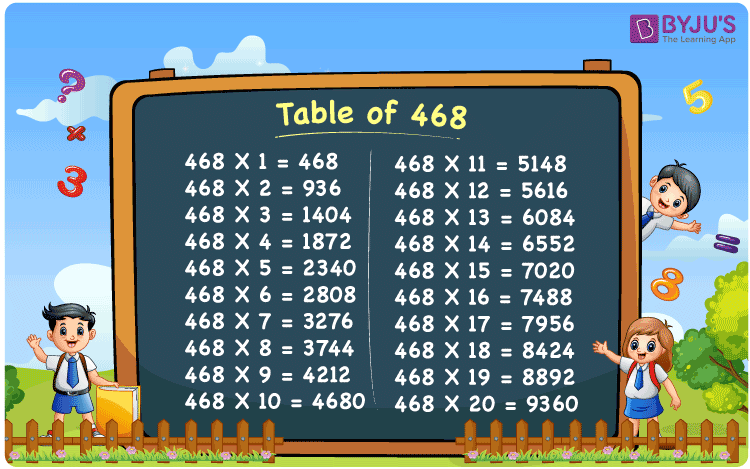Checkout JEE MAINS 2022 Question Paper Analysis : Checkout JEE MAINS 2022 Question Paper Analysis :

# Table of 468

The table of 468 is provided here to help students verify the table of 468 values up to 20 times. In this page, we have presented the tabular representation of the 468 times table. To obtain the multiplication table of 468, download the PDF, which is also available on our page. Students can utilise the table of 468 chart as a flashcard to help them learn and recall the values faster.

## Table of 468 Chart## What is the 468 Times Table?

The repeated addition and multiplication table of 468 is provided below.

 468×1 = 468 468 468×2 = 936 468 + 468 = 936 468×3 = 1404 468 + 468 + 468 = 1404 468×4 = 1872 468 + 468 + 468 + 468 = 1872 468×5 = 2340 468 + 468 + 468 + 468 + 468 = 2340 468×6 = 2808 468 + 468 + 468 + 468 + 468 + 468 = 2808 468×7 = 3276 468 + 468 + 468 + 468 + 468 + 468 + 468 = 3276 468×8 = 3744 468 + 468 + 468 + 468 + 468 + 468 + 468 + 468 = 3744 468×9 = 4212 468 + 468 + 468 + 468 + 468 + 468 + 468 + 468 + 468 = 4212 468×10 = 4680 468 + 468 + 468 + 468 + 468 + 468 + 468 + 468 + 468 + 468 = 4680

## Multiplication Table of 468

The first 20 multiples of 468 are displayed in the table below.

 468 × 1 = 468 468 × 2 = 936 468 × 3 = 1404 468 × 4 = 1872 468 × 5 = 2340 468 × 6 = 2808 468 × 7 = 3276 468 × 8 = 3744 468 × 9 = 4212 468 × 10 = 4680 468 × 11 = 5148 468 × 12 = 5616 468 × 13 = 6084 468 × 14 = 6552 468 × 15 = 7020 468 × 16 = 7488 468 × 17 = 7956 468 × 18 = 8424 468 × 19 = 8892 468 × 20 = 9360

## Solved Example on the Table of 468

Example:

Determine the unknown value: 468 × ____ = 3276

Solution:

Let the missing value be x.

Hence, 468x = 3276

x = 3276/468

x = 7

Hence, the unknown number is 7.

I.e., 468 × 7 = 3276.

## Frequently Asked Questions on the Table of 468

### What is the table of 468?

The table of 468 is the multiplication table, which is obtained by multiplying 468 with natural numbers.

### Write the 468 times table.

468×1 = 468, 468×2 = 936, 468×3 = 1404, 468×4 = 1872, 468×5 = 2340, 468×6 = 2808, 468×7 = 3276, 468×8 = 3744, 468×9 = 4212, 468×10 = 4680.

### How many times 468 is 4680?

10 times 468 is 4680. I.e., 468 × 10 = 4680.